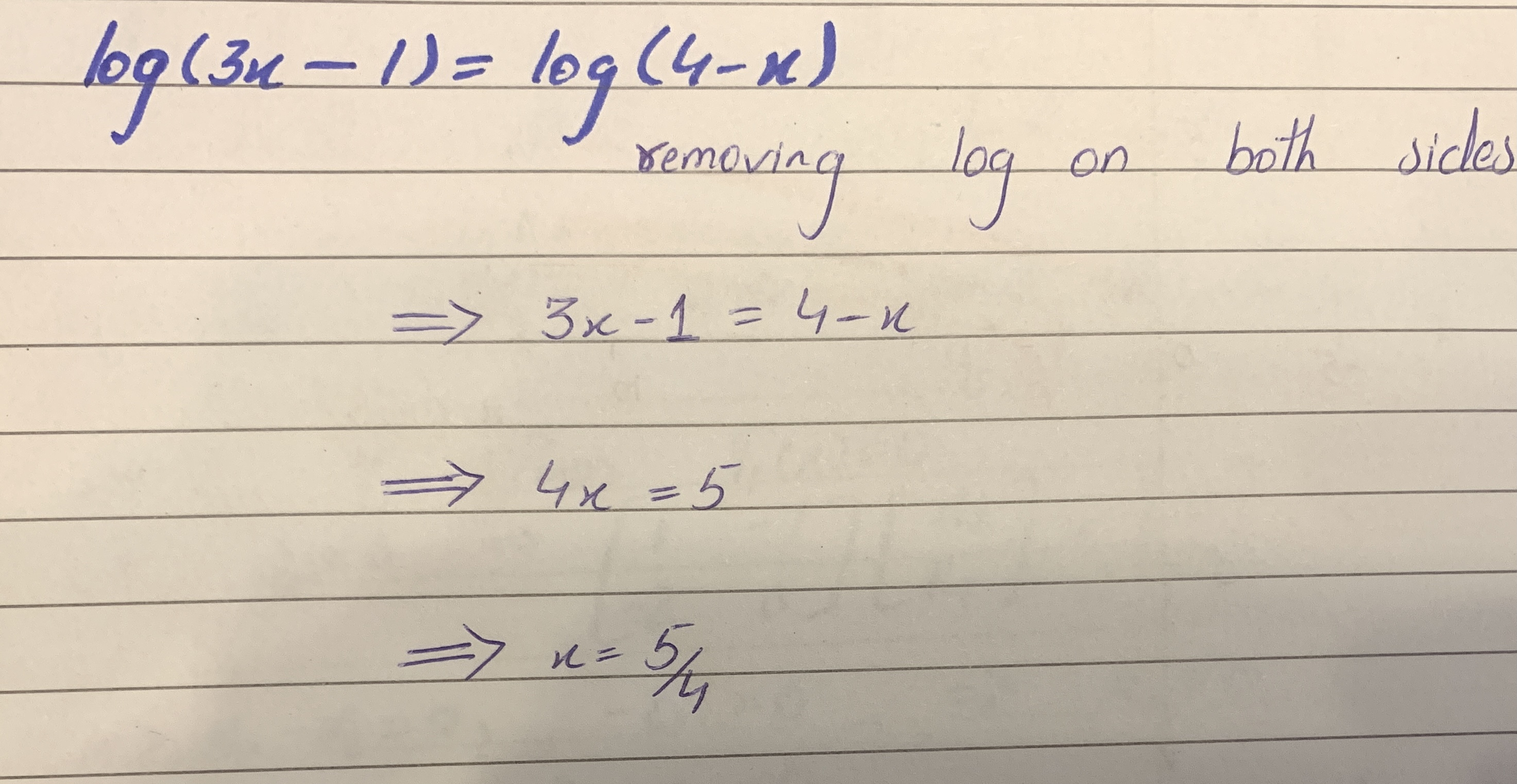# Solve the following equation: log(3x-1)=log(4-x)sagnuhh 2021-01-19 Answered
Solve the following equation:
$log\left(3x-1\right)=log\left(4-x\right)$
You can still ask an expert for help

• Questions are typically answered in as fast as 30 minutes

Solve your problem for the price of one coffee

• Math expert for every subject
• Pay only if we can solve itNola Robson

See the solution -